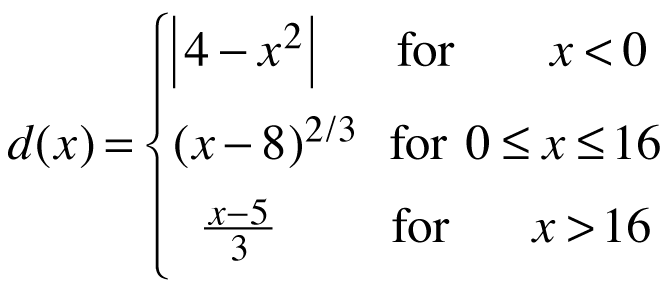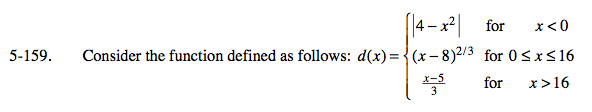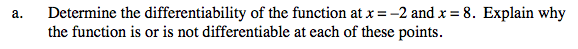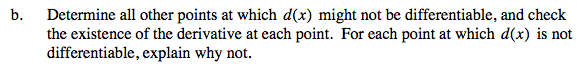### Home > CALC > Chapter 5 > Lesson 5.5.1 > Problem5-159

5-159.
1. Consider the function defined as follows:Homework Help ✎

1. Determine the differentiability of the function at x = −2 and x = 8. Explain why the function is or is not differentiable at each of these points.

2. Determine all other points at which d(x) might not be differentiable, and check the existence of the derivative at each point. For each point at which d(x) is not differentiable, explain why not.When investigating whether a function is differentiable at a point, there are two things to consider:
1. Differentiability implies continuity. So you need to make sure that d(x) is continuous before you ask if it is differentiable. (Remember, there are 3 conditions of continuity must be explored.)
2. Is the derivative continuous? In other words, does the slope from the right agree with the slope from the left, and agree with the actual slope? Also, are those slopes finite?Check boundary points x = 0 and x = 16.